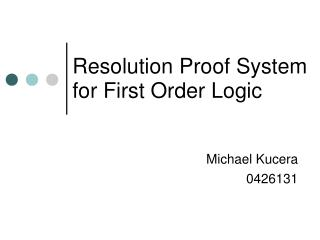Download PresentationResolution Proof System for First Order Logic# Resolution Proof System for First Order Logic - PowerPoint PPT Presentation

Download Presentation##### Resolution Proof System for First Order Logic

Download Policy: Content on the Website is provided to you AS IS for your information and personal use and may not be sold / licensed / shared on other websites without getting consent from its author. While downloading, if for some reason you are not able to download a presentation, the publisher may have deleted the file from their server.

- - - - - - - - - - - - - - - - - - - - - - - - - - - E N D - - - - - - - - - - - - - - - - - - - - - - - - - - -
##### Presentation Transcript

1. Michael Kucera 0426131 Resolution Proof System for First Order Logic

2. Resolution for propositional logic • Ground (propositional) resolution is a single sound inference rule that is applied to clauses. • A clause is a disjunction of literals. • Example, (p ¬q r) is a clause. • Resolution: (α1 p), (α2 ¬p) (α1 α2) • Example, (¬p  q), (¬q r) (¬p r)

3. Clausal form • In order to use resolution for first order logic the formulas must be converted into clausal form. • A clause is a disjunction of literals with no literal appearing twice, no existential quantifiers and all universal quantifiers are at the left. • Ex, xyz( P(x,y) ¬Q(y) R(z,y) ) • The empty clause, denoted by , is unsatisfiable. • A unit clause contains just one literal. • The universal quantifiers may be omitted.

4. Skolemization • Process for removing existential quantifiers. • Delete each existential quantifier, then replace the resulting free variables by terms referred to as Skolem functions. • xy P(x,y) becomes y P(c, y) • xy P(x,y) becomes x P(x, f(x)) • Skolemization preserves satisfiability.

5. Conversion to clausal form • Eliminate and • Move ¬ inwards • Rename variables if two quantifiers have the same bound variable name. • Eliminate existential quantifiers using Skolem functions. • Move all universal quantifiers to the left (prenex normal form). • Transform the matrix into conjunctive normal form.

6. Conversion to clausal form • This produces the form x1x2...xm(C1 ... Cn) • Where C1 ... Cn are clauses. • Each step preserves logical equivalence except Skolemization which only preserves satisfiability.

7. Unification • A substitution, denoted by θ, is an assignment from variables to terms. • Example: if θ is {x → g(y)} and E is P(x,w,f(x)) then Eθ is P(g(y),w,f(g(y))) • Unification is the process of replacing the variables in expressions by terms to make the modified expressions identical to each other. • Example: Let E1=P(x,a) , E2=P(y,a) , θ = {y→x} Then E1θ = E2θ = P(x,a) • I the above example θ is the most general unifier (mgu)

8. Resolution for FOL (α1L)θ, (α2¬L)θ (α1α2)θ • θ is the most general unifier for L. • The result of resolution is called the resolvent. • There may be more than one possible reslolvent. • The resolvent of two complementary unit clauses is the empty clause.

9. Resolution for first order logic • Resolution is refutationally complete (with factoring). • Factoring is an additional rule that allows you to remove duplicate literals within a clause. • Proving ׀= A is equivalent to proving that  {¬A} is unsatisfiable. • A is called the goal. • In a resolution proof you negate the goal and add it to the set of hypotheses, then apply resolution until the empty clause is produced.

10. Clausal Form ¬dog(x) animal(x) dog(fido) ¬animal(y) die(y) Negate the goal ¬die(fido) Example Hypothesies x (dog(x) animal(x)) dog(fido) y (animal(y)  die(y)) Conclusion die(fido)

11. Example ¬dog(x)  animal(x) ¬animal(y)  die(y) {x → y} {x dog(fido) ¬dog(y)  die(y) {y → fido} ¬die(fido) die(fido) 

12. Automated theorem proving • Resolution is used in automated theorem provers like Otter. • Don't have to choose which rule of inference to use because there is only one. • No axiom schemata to instantiate. • Many refinements such as Hyper-resolution and Lock resolution. • Search strategies remove redundancy of preforming all posible deduction sequences.